日前，北斗航天汽车（北京）有限公司（以下简称北斗航天汽车）与金龙联合汽车工业（苏州）有限公司（以下简称苏州金龙汽车）联合出品的CLV550X纯电动物流车第一批次车辆生产下线。根据双方的合作协议，依托苏州金龙汽车的生产能力和北斗航天汽车的核心“三电”技术，未来双方将研发生产更多的新能源车型。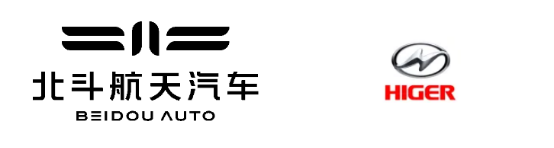这是北斗航天汽车继与一汽吉林汽车联合生产CLV440纯电动物流车后，与苏州金龙汽车联合生产的最新款纯电动城市物流车CLV550X，品牌为CLV，即“美好生活”的意思，表达出一种向往美好的人生态度和舒适惬意的生活体验，契合了北斗航天汽车人对未来纯电动城市物流车业态的美好向往。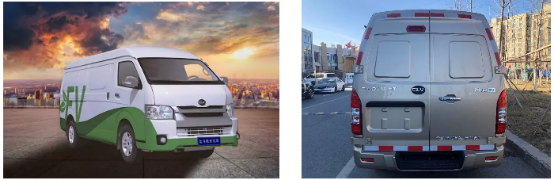CLV550X示意图

CLV550X纯电动物流车高度融合了北斗航天汽车核心“三电”技术，精选高品质、高能量密度和高安全性动力电池，具有高电量、长续航的特性；创新集成电机与二级减速器，降低动力系统总成重量，最大功率达140kw，峰值扭矩可达320N.m，车辆爬坡有劲，加速快，再多货物也能从容应对；车身高度2220mm，能自由出入地下停车场及车库；后对开门设计，满足叉车托盘对接作业；1150mm侧拉门设计，方便货物进出。超大的载货空间、超强的运载能力、纯电动无污染，是城市物流运输的最佳选择。同时，CLV550X纯电动物流车基于北斗高精度定位技术，搭载了北斗车联网平台、北斗高精度车载导航等，使车辆更智能，运行更高效。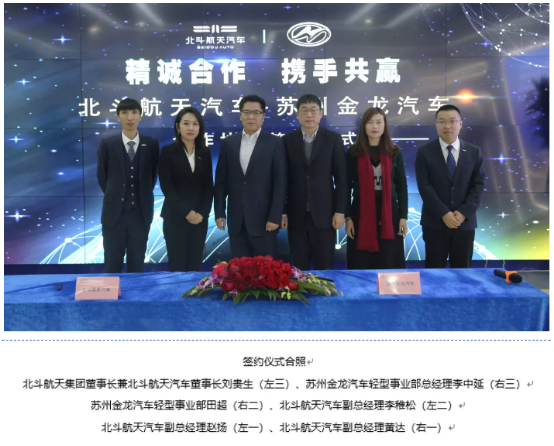苏州金龙汽车是国家汽车整车出口基地企业，是中国客车行业发展最快的企业以及中国企业信息化100强，具有年产25000台大中型客车及底盘的能力，下辖博士后科研工作站、江苏省级企业技术中心、新型高速客车研发中心、园区现代化客车生产基地。其生产的海格客车荣获“中国名牌”、“国家出口免验产品”等称号，品牌价值跻身“中国500最具价值品牌”榜。苏州金龙汽车秉承"智赢未来"的品牌主张，在客车行业首家研发并推出”G-BOS智慧运营系统",实现从客车制造商向客车运营管理整体方案提供商的转变，以打造"安全、舒适、高性价比"的智慧客车为己任，成为我国智慧安全客车的领航者。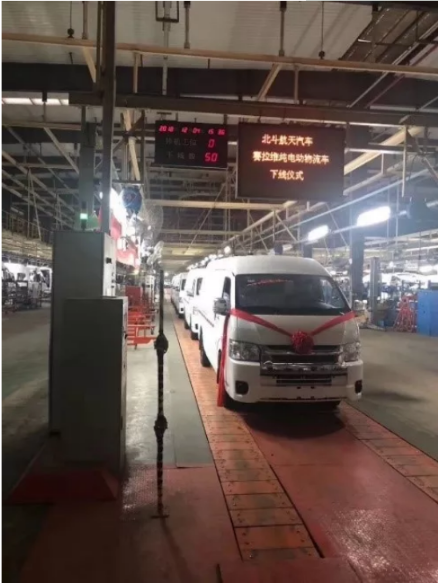CLV550X纯电动物流车正式下线

北斗航天汽车是北斗航天集团旗下子公司，主要从事新能源车的新材料、新技术的研究开发。目前已拥有“电池、电机、电控”三大核心的自主技术专利近300件，其中发明专利达30%，形成了从“三电”方案解决、“三电”产品提供到整车装配制造的生态体系。通过与业内优秀资源合作，北斗航天汽车致力于打造世界级新能源车科技创新中心。

以此次CLV550X纯电动物流车的下线为契机，北斗航天汽车与苏州金龙汽车将加大合作力度，从纯电动物流车的研发生产到市场导入，强强联手共同打造绿色物流产业生态闭环。

##### 相关资讯

|分享

•已阅！握爪
•我手滑为你点赞
•128个赞！
•不明觉厉
•阅后既醉
•有钱！任性
•照片太美，我不敢看
•干货！杠杠的
•高大上
•膜拜中！
•请接受我的膝盖
•猫哥/猫妹么么哒
•神吐槽
•我只笑笑不说话
•我想静静
•窒息
##### 资讯相关车系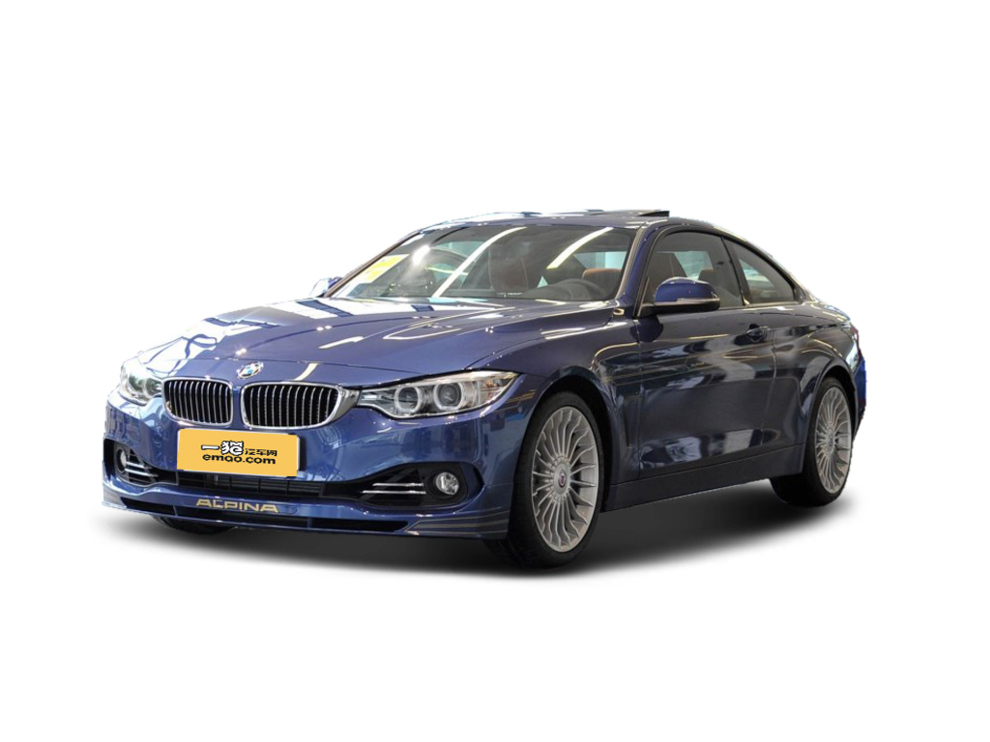##### 热度排行• 阿斯顿·马丁
• 奥迪
• 阿尔法罗密欧
• ALPINA

• 巴博斯
• 宝骏
• 宝马
• 保时捷
• 北汽制造
• 奔驰
• 奔腾
• 本田
• 比亚迪
• 标致
• 别克
• 宾利
• 布加迪
• 北汽威旺
• 北京
• 北汽绅宝
• 北汽幻速
• 北汽新能源
• 宝沃
• 比速汽车
• 北汽道达

• 昌河
• 长安
• 长城
• 长安商用
• 成功汽车
• 长江EV
• 长安轻车型

• 大众
• 道奇
• 东风
• 东风风神
• 东风小康
• 东南
• DS
• 东风风行
• 东风风度
• 东风风光

• 法拉利
• 菲亚特
• 丰田
• 福迪
• 福特
• 福田汽车
• 福汽启腾
• 风诺

• GMC
• 广汽传祺
• 广汽吉奥
• 观致

• 哈飞
• 海格
• 海马
• 华泰
• 黄海
• 恒天
• 红旗
• 哈弗
• 华颂
• 华凯
• 华泰新能源
• 汉腾汽车

• Jeep
• 江淮
• 江铃
• 捷豹
• 金杯
• 九龙
• 吉利汽车
• 金旅
• 金龙
• 江铃集团轻汽
• 江铃集团新能源
• 君马汽车
• 捷途

• 开瑞
• 凯迪拉克
• 科尼赛克
• 克莱斯勒
• KTM
• 卡威
• 凯翼
• 康迪
• 康迪电动汽车集团

• 猎豹汽车
• 兰博基尼
• 劳斯莱斯
• 雷克萨斯
• 雷诺
• 理念
• 力帆
• 莲花汽车
• 林肯
• 铃木
• 陆风
• 路虎
• 路特斯
• 领志
• 领克
• 零跑汽车

• MG
• MINI
• 马自达
• 玛莎拉蒂
• 迈凯伦
• 摩根

• 纳智捷

• 讴歌
• 欧宝
• 欧朗
• 欧拉
• 欧尚汽车

• 帕加尼

• 奇瑞
• 启辰
• 起亚
• 前途
• 庆铃汽车

• 日产
• 荣威
• 瑞麒汽车
• 如虎
• 瑞驰

• 上汽大通
• smart
• 三菱
• 双环
• 双龙
• 斯巴鲁
• 斯柯达
• 萨博
• 思铭
• 赛麟
• SWM斯威汽车

• TESLA
• 泰卡特
• 腾势

• 威麟
• 威兹曼
• 沃尔沃
• 五菱汽车
• 五十铃
• 潍柴英致
• WEY
• 蔚来
• 威马汽车

• 现代
• 雪佛兰
• 雪铁龙
• 西雅特
• 新特汽车
• 小鹏汽车
• 新宝骏

• 野马汽车
• 一汽
• 依维柯
• 英菲尼迪
• 永源
• 驭胜

• 中华
• 中兴
• 众泰
• 知豆
• 之诺
• 正道汽车
• A
• B
• C
• D
• E
• F
• G
• H
• I
• J
• K
• L
• M
• N
• O
• P
• Q
• R
• S
• T
• U
• V
• W
• X
• Y
• Z

• 阿斯顿·马丁
• 奥迪
• 阿尔法罗密欧
• ALPINA

• 巴博斯
• 宝骏
• 宝马
• 保时捷
• 北汽制造
• 奔驰
• 奔腾
• 本田
• 比亚迪
• 标致
• 别克
• 宾利
• 布加迪
• 北汽威旺
• 北京
• 北汽绅宝
• 北汽幻速
• 北汽新能源
• 宝沃
• 比速汽车
• 北汽道达

• 昌河
• 长安
• 长城
• 长安商用
• 成功汽车
• 长江EV
• 长安轻车型

• 大众
• 道奇
• 东风
• 东风风神
• 东风小康
• 东南
• DS
• 东风风行
• 东风风度
• 东风风光

• 法拉利
• 菲亚特
• 丰田
• 福迪
• 福特
• 福田汽车
• 福汽启腾
• 风诺

• GMC
• 广汽传祺
• 广汽吉奥
• 观致

• 哈飞
• 海格
• 海马
• 华泰
• 黄海
• 恒天
• 红旗
• 哈弗
• 华颂
• 华凯
• 华泰新能源
• 汉腾汽车

• Jeep
• 江淮
• 江铃
• 捷豹
• 金杯
• 九龙
• 吉利汽车
• 金旅
• 金龙
• 江铃集团轻汽
• 江铃集团新能源
• 君马汽车
• 捷途

• 开瑞
• 凯迪拉克
• 科尼赛克
• 克莱斯勒
• KTM
• 卡威
• 凯翼
• 康迪
• 康迪电动汽车集团

• 猎豹汽车
• 兰博基尼
• 劳斯莱斯
• 雷克萨斯
• 雷诺
• 理念
• 力帆
• 莲花汽车
• 林肯
• 铃木
• 陆风
• 路虎
• 路特斯
• 领志
• 领克
• 零跑汽车

• MG
• MINI
• 马自达
• 玛莎拉蒂
• 迈凯伦
• 摩根

• 纳智捷

• 讴歌
• 欧宝
• 欧朗
• 欧拉
• 欧尚汽车

• 帕加尼

• 奇瑞
• 启辰
• 起亚
• 前途
• 庆铃汽车

• 日产
• 荣威
• 瑞麒汽车
• 如虎
• 瑞驰

• 上汽大通
• smart
• 三菱
• 双环
• 双龙
• 斯巴鲁
• 斯柯达
• 萨博
• 思铭
• 赛麟
• SWM斯威汽车

• TESLA
• 泰卡特
• 腾势

• 威麟
• 威兹曼
• 沃尔沃
• 五菱汽车
• 五十铃
• 潍柴英致
• WEY
• 蔚来
• 威马汽车

• 现代
• 雪佛兰
• 雪铁龙
• 西雅特
• 新特汽车
• 小鹏汽车
• 新宝骏

• 野马汽车
• 一汽
• 依维柯
• 英菲尼迪
• 永源
• 驭胜

• 中华
• 中兴
• 众泰
• 知豆
• 之诺
• 正道汽车
• A
• B
• C
• D
• E
• F
• G
• H
• I
• J
• K
• L
• M
• N
• O
• P
• Q
• R
• S
• T
• U
• V
• W
• X
• Y
• Z

• 阿斯顿·马丁
• 奥迪
• 阿尔法罗密欧
• ALPINA

• 巴博斯
• 宝骏
• 宝马
• 保时捷
• 北汽制造
• 奔驰
• 奔腾
• 本田
• 比亚迪
• 标致
• 别克
• 宾利
• 布加迪
• 北汽威旺
• 北京
• 北汽绅宝
• 北汽幻速
• 北汽新能源
• 宝沃
• 比速汽车
• 北汽道达

• 昌河
• 长安
• 长城
• 长安商用
• 成功汽车
• 长江EV
• 长安轻车型

• 大众
• 道奇
• 东风
• 东风风神
• 东风小康
• 东南
• DS
• 东风风行
• 东风风度
• 东风风光

• 法拉利
• 菲亚特
• 丰田
• 福迪
• 福特
• 福田汽车
• 福汽启腾
• 风诺

• GMC
• 广汽传祺
• 广汽吉奥
• 观致

• 哈飞
• 海格
• 海马
• 华泰
• 黄海
• 恒天
• 红旗
• 哈弗
• 华颂
• 华凯
• 华泰新能源
• 汉腾汽车

• Jeep
• 江淮
• 江铃
• 捷豹
• 金杯
• 九龙
• 吉利汽车
• 金旅
• 金龙
• 江铃集团轻汽
• 江铃集团新能源
• 君马汽车
• 捷途

• 开瑞
• 凯迪拉克
• 科尼赛克
• 克莱斯勒
• KTM
• 卡威
• 凯翼
• 康迪
• 康迪电动汽车集团

• 猎豹汽车
• 兰博基尼
• 劳斯莱斯
• 雷克萨斯
• 雷诺
• 理念
• 力帆
• 莲花汽车
• 林肯
• 铃木
• 陆风
• 路虎
• 路特斯
• 领志
• 领克
• 零跑汽车

• MG
• MINI
• 马自达
• 玛莎拉蒂
• 迈凯伦
• 摩根

• 纳智捷

• 讴歌
• 欧宝
• 欧朗
• 欧拉
• 欧尚汽车

• 帕加尼

• 奇瑞
• 启辰
• 起亚
• 前途
• 庆铃汽车

• 日产
• 荣威
• 瑞麒汽车
• 如虎
• 瑞驰

• 上汽大通
• smart
• 三菱
• 双环
• 双龙
• 斯巴鲁
• 斯柯达
• 萨博
• 思铭
• 赛麟
• SWM斯威汽车

• TESLA
• 泰卡特
• 腾势

• 威麟
• 威兹曼
• 沃尔沃
• 五菱汽车
• 五十铃
• 潍柴英致
• WEY
• 蔚来
• 威马汽车

• 现代
• 雪佛兰
• 雪铁龙
• 西雅特
• 新特汽车
• 小鹏汽车
• 新宝骏

• 野马汽车
• 一汽
• 依维柯
• 英菲尼迪
• 永源
• 驭胜

• 中华
• 中兴
• 众泰
• 知豆
• 之诺
• 正道汽车
• A
• B
• C
• D
• E
• F
• G
• H
• I
• J
• K
• L
• M
• N
• O
• P
• Q
• R
• S
• T
• U
• V
• W
• X
• Y
• Z